## mimeTeX: LaTeX Math for Wordpress

This Wordpress plugin allows you to embed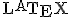$\LaTeX$ math formulas into your posts without having LaTeX installed at your server. Just like this: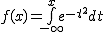$\normalsize f(x)=\int\limits_{-\infty}^x~e^{-t^2}dt$

It was originally developed by Anlak.

The plugin just downloads the image from the public cgi script in Forkosh and copies it to your wp-content/cache.

I modified the plugin to correct two problems: the cgi script url has changed and there were some problems with tags and characters encoding. For the first issue I placed a script in my page that will keep the url updated without changes in the plugin code.

Installation procedure:

1. Copy both file to your “/wp-content/plugins”-folder.
2. Create the folder “/wp-content/cache” and chmod it to 777.
3. Go to the administration interface. In Plugins, activate it.

Enjoy!

Some other examples:$\normalsize f(x)=\int\limits_{-\infty}^x~e^{-t^2}dt$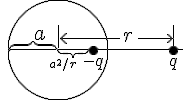$\Large\hspace{5}\unitlength{1} \picture(175,100){~(50,50){\circle(100)} (1,50){\overbrace{\line(46)}^{4\;\;a}} (52,50){\line(125)}~(50,52;115;2){\mid}~(52,55){\longleftar} (130,56){\longrightar}~(116,58){r}~(c85,50;80;2){\bullet} (c85,36){3-q}~(c165,36){3q} (42,29){\underbrace{\line(32)}_{1a^2/r\;\;\;}}~}$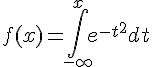$\Large f(x)=\int_{-\infty}^x e^{-t^2}dt$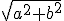$\sqrt{a^2 + b^2}$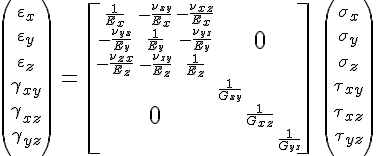$\normalsize \left(\large\begin{array}{GC+23} \varepsilon_x\\\varepsilon_y\\\varepsilon_z\\\gamma_{xy}\\ \gamma_{xz}\\\gamma_{yz}\end{array}\right)\ {\Large=} \ \left[\begin{array}{CC} \begin{array}\frac1{E_{\fs{+1}x}} &-\frac{\nu_{xy}}{E_{\fs{+1}x}} &-\frac{\nu_{\fs{+1}xz}}{E_{\fs{+1}x}}\\ -\frac{\nu_{yx}}{E_y}&\frac1{E_{y}}&-\frac{\nu_{yz}}{E_y}\\ -\frac{\nu_{\fs{+1}zx}}{E_{\fs{+1}z}}& -\frac{\nu_{zy}}{E_{\fs{+1}z}} &\frac1{E_{\fs{+1}z}}\end{array} & {\LARGE 0} \\ {\LARGE 0} & \begin{array}\frac1{G_{xy}}&&\\ &\frac1{G_{\fs{+1}xz}}&\\&&\frac1{G_{yz}}\end{array} \end{array}\right] \ \left(\large\begin{array} \sigma_x\\\sigma_y\\\sigma_z\\\tau_{xy}\\\tau_{xz}\\\tau_{yz} \end{array}\right)$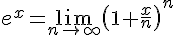$\Large e^x=\lim_{n\to\infty} \left(1+\frac~xn\right)^n$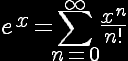$\reverse\opaque \LARGE e^x=\sum_{n=0}^\infty\frac{x^n}{n!}$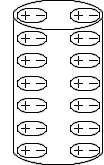$\small\hspace{10}\unitlength{.75} \picture(120,220){~(60,200){\circle(120,40)}~(0,20){\line(0,180)} (5,189;0,-30){\pict(110,20){(c20,10;70;2){ \pict(40,20){(20,10){\circle(40,20)}(c10,10)+(c30,10)-}}~}~} (119,20){\line(0,180)}~(60,20){\circle(120,40;34)}}$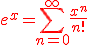$\red\large\displaystyle e^x=\sum_{n=0}^\infty\frac{x^n}{n!}$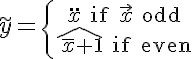$\LARGE\tilde y=\left\{ {\ddot x\text{ if \vec x odd}\atop\hat{\,\bar x+1}\text{ if even}}\right.$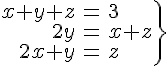$\Large\left.\begin{eqnarray} x+y+z&=&3\\2y&=&x+z\\2x+y&=&z\end{eqnarray}\right\}$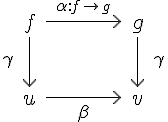$\Large\begin{array}{rccclBCB} &f&\longr^{\alpha:{\normalsize f\rightar~g}}&g\\ \large\gamma&\longd&&\longd&\large\gamma\\ &u&\longr_\beta&v\end{array}$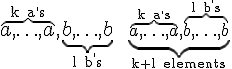$\Large\overbrace{a,\ldots,a}^{\text{k a^,s}}, \underbrace{b,\ldots,b}_{\text{l b^,s}}\hspace{10} \large\underbrace{\overbrace{a,\ldots,a}^{\text{k a^,s}}, \overbrace{b,\ldots,b}^{\text{l b^,s}}}_{\text{k+l elements}}$

### One Response to “mimeTeX: LaTeX Math for Wordpress”

1. İsmail Arı Says:

Thanks for the great plugin!